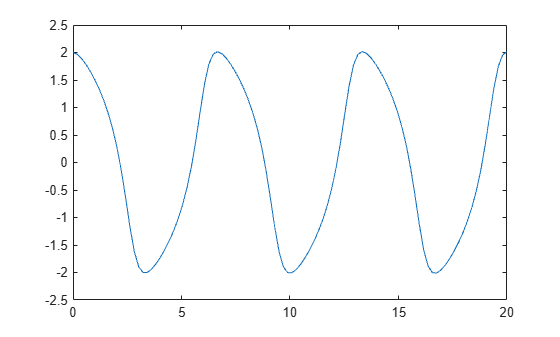deval

Evaluate differential equation solution structure

Description

example

y = deval(sol,x) and y = deval(x,sol) evaluate the solution sol of a differential equation problem at the points contained in x.

example

y = deval(___,idx) returns only the solution components with indices listed in the vector idx. You can use either of the previously listed input argument combinations.

example

[y,yp] = deval(___) also returns yp, which is the first derivative of the numeric solution produced by the solver.

Examples

collapse all

This example solves the DDE equation y' = ddex1de(t,y,Z) using dde23, then plots the solution.

Solve the system using dde23.

sol = dde23(@ddex1de, [1 0.2], @ddex1hist, [0 5]);

Evaluate the solution at 100 points in the interval [0 5].

x = linspace(0,5);
y = deval(sol,x);

Plot the solution.

plot(x,y)This example solves the system y' = vdp1(t,y) using ode45, then plots the first component of the solution.

Solve the system using ode45.

sol = ode45(@vdp1, [0 20], [2 0]);

Evaluate the first component of the solution at 100 points in the interval [0 20].

x = linspace(0,20,100);
y = deval(sol,x,1);

Plot the solution.

plot(x,y)Solve the simple ODE y' = t^2 with initial condition y0 = 0 in the interval $\left[0,3\right]$ using ode23.

sol = ode23(@(t,y) t^2, [0 3], 0);

Evaluate the solution at seven points. The solution structure sol contains an interpolating function that deval uses to produce a continuous solution at these points. Specify a second output argument with deval to also return the derivative of the interpolating function at the specified points.

x = linspace(0,3,7);
[y,yp] = deval(sol,x)
y = 1×7

0    0.0417    0.3333    1.1250    2.6667    5.2083    9.0000

yp = 1×7

0    0.2500    1.0000    2.2500    4.0000    6.2500    9.0000

Input Arguments

collapse all

Solution structure, specified as a structure returned by one of these differential equation solvers.

• Initial value problem solver — ode45, ode23, ode113, ode15s, ode23s, ode23t, ode23tb, ode15i

• Delay differential equations solver — dde23, ddesd, or ddensd

• Boundary value problem solver — bvp4c or bvp5c

Example: sol = ode45(@myode,tspan,y0)

Data Types: struct

Evaluation points, specified as a vector. x specifies the points at which you want the value of the solution. The elements of x must be contained in the original integration interval, [sol.x(1) sol.x(end)]. For each index i, the solution y(:,i) corresponds to x(i).

Example: 0:0.1:1

Example: [2 3 5 8]

Data Types: single | double

Solution components to return, specified as a vector. Use this input when you are only interested in certain components of the solution.

Example: y = deval(sol,x,[2 3]) returns only the second and third solution components.

Data Types: single | double

Output Arguments

collapse all

Interpolated solution, returned as a vector or matrix. The number of rows in y is equal to the number of solution components being returned.

For multipoint boundary value problems, the solution obtained by bvp4c or bvp5c might be discontinuous at the interfaces. For an interface point xc, the deval function returns the average of the limits from the left and right of xc. To get the limit values, set the value of x to be slightly larger or smaller than xc.

Derivative of continuous solution produced by sol, returned as a vector or matrix. yp is the same size as y and indicates the slope of the interpolating function used by sol at each solution point in y.